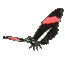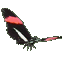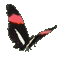Graphs of Exponential Functions     Math Help     Game Tips:

- The relations y=2x, y=3x, and y=4x are examples of exponential functions.

- A 'positive constant base raised to a variable exponent' = (constant)(variable) is an 'Exponential function'.
- In general, an 'Exponential function' is represented by the formula y=ax where a>0.
Notice that 'a' cannot be '1' ,
and when integer a>0, the graph of y=ax passes through the point (0,1).

- The relations y=3(2x), y=4(6x), and y=2(3x) are examples of exponential functions with a front multiplier.
- In general, a 'Constant times an Exponential function' can be represented by a formula such as y=k(ax).
For integer a>0, the graph of y=k(ax) passes through the point (0,k).

- In this game, 'k' is an integer from -3 to 3 and 'a' is an integer from 2 to 5.

- Notice y=2x has graph points such as (-1,1/2), (0,1), (1,2), and (2,4)... ,
while a relation such as y=2(3x) has graph points such as (-1,2/3), (0,2), (1,6), and (2,18)... .

- There are many applications of exponential functions. For example, exponentials are used in formulas
for compound interest, for geometric growth patterns [ax], and for radioactive decay patterns [a-x].

- Your Game Score is reduced by the number of butterfly hits.

- To slow the game speed repeat tap/click on the word Slider.
- To increase the game speed repeat tap/click on the word Math.
- Speed can also be adjusted with a keyboard's - and + keys.

- Refresh/Reload the web page to restart the game.